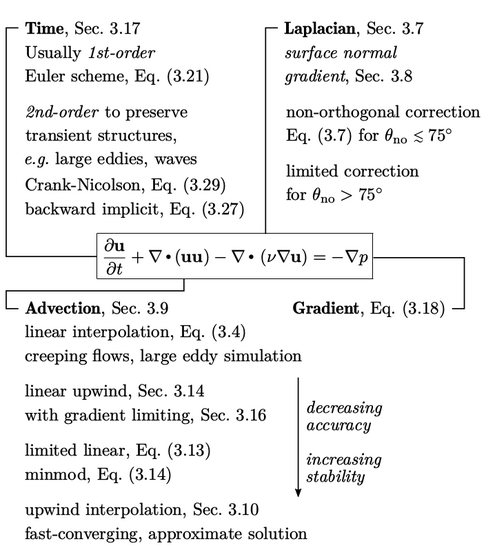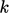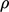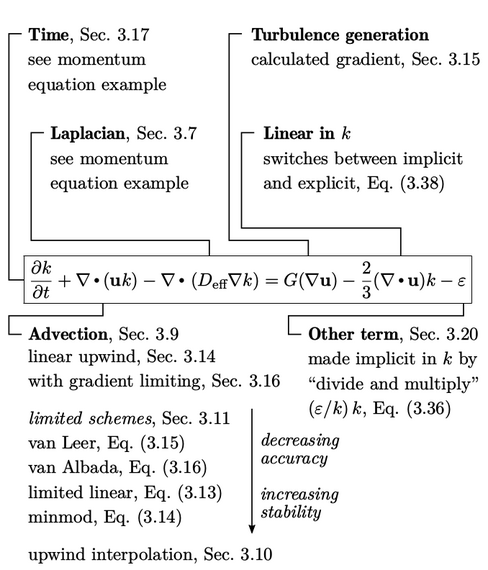## 3.23Recommended discretisation schemes

The following ﬁgures provide a summary and recommendations of discretisation schemes for diﬀerent equations. We start with momentum conservation Eq. (2.49 ), described in Sec. 2.13 and Sec. 2.14 , for which accuracy is the main consideration in scheme selection.### Equation for a bounded property

The discretisation of an equation for a bounded scalar ﬁeld is illustrated using theequation from theturbulence model (assuming constant). Schemes are selected with an emphasis on boundedness.Notes on CFD: General Principles - 3.23 Recommended discretisation schemes